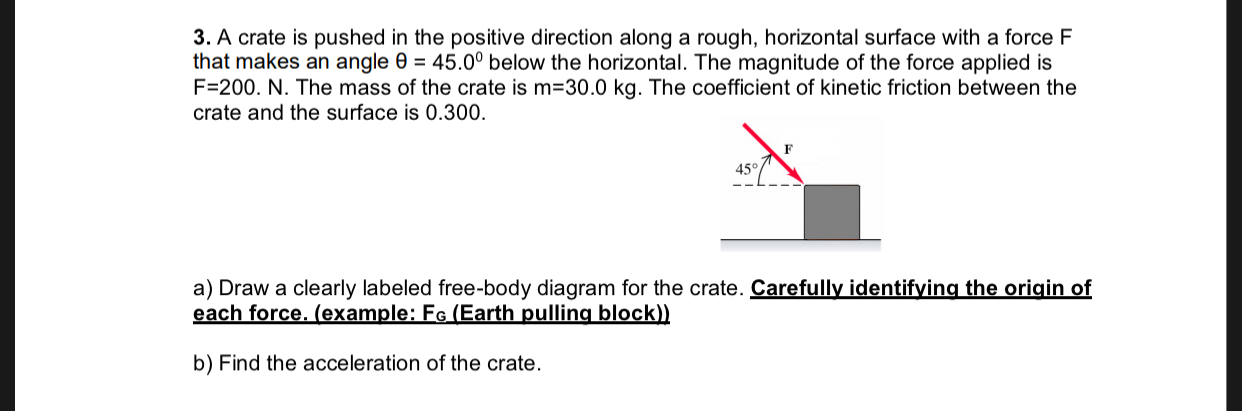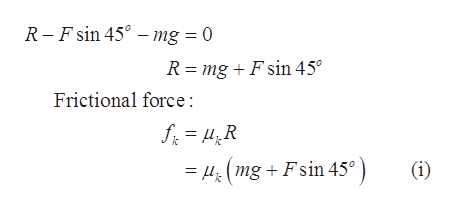# 3. A crate is pushed in the positive direction along a rough, horizontal surface with a force Fthat makes an angle e 45.00 below the horizontal. The magnitude of the force applied isF-200. N. The mass of the crate is m 30.0 kg. The coefficient of kinetic friction between thecrate and the surface is 0.300.a) Draw a clearly labeled free-body diagram for the crate. Carefully identifying the origin ofeach force. (example: Fe (Earth pulling block))b) Find the acceleration of the crate

Question
2 views

I need help on thathelp_outlineImage Transcriptionclose3. A crate is pushed in the positive direction along a rough, horizontal surface with a force F that makes an angle e 45.00 below the horizontal. The magnitude of the force applied is F-200. N. The mass of the crate is m 30.0 kg. The coefficient of kinetic friction between the crate and the surface is 0.300. a) Draw a clearly labeled free-body diagram for the crate. Carefully identifying the origin of each force. (example: Fe (Earth pulling block)) b) Find the acceleration of the crate fullscreen
check_circle

Step 1

Given values:

F = 200 N

m = 30.0 kg

the coefficient of kinetic friction between the crate and the surface = 0.3

Step 2

(a)

The free diagram for the crate:

Step 3

(b)

Applying the Newton’s second law Along vertical component:

There is ...help_outlineImage TranscriptioncloseR-F sin 45 - mg = 0 _ R mgFsin 45° Frictional force: mgFsin 45°) (i) fullscreen

### Want to see the full answer?

See Solution

#### Want to see this answer and more?

Solutions are written by subject experts who are available 24/7. Questions are typically answered within 1 hour.*

See Solution
*Response times may vary by subject and question.
Tagged in

### Other